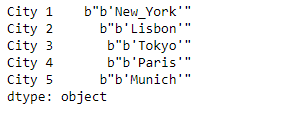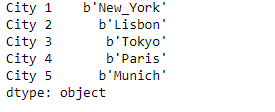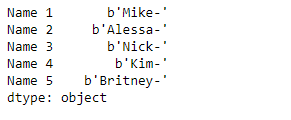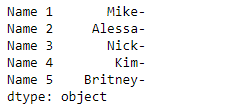# Python | Pandas Series.str.decode()

• Last Updated : 27 Mar, 2019

`Series.str` can be used to access the values of the series as strings and apply several methods to it. Pandas` Series.str.decode()` function is used to decode character string in the Series/Index using indicated encoding. This function is equivalent to `str.decode()` in python2 and `bytes.decode()` in python3.

Syntax: Series.str.decode(encoding, errors=’strict’)

Parameter :
encoding : str
errors : str, optional

Returns : decoded Series/Index of objects

Example #1: Use `Series.str.decode()` function to decode the character strings in the underlying data of the given series object. Use ‘UTF-8’ encoding method.

 `# importing pandas as pd``import` `pandas as pd`` ` `# Creating the Series``sr ``=` `pd.Series([b``"b'New_York'"``, b``"b'Lisbon'"``, b``"b'Tokyo'"``, b``"b'Paris'"``, b``"b'Munich'"``])`` ` `# Creating the index``idx ``=` `[``'City 1'``, ``'City 2'``, ``'City 3'``, ``'City 4'``, ``'City 5'``]`` ` `# set the index``sr.index ``=` `idx`` ` `# Print the series``print``(sr)`

Output :Now we will use `Series.str.decode()` function to decode the character strings in the underlying data of the given series object.

 `# use 'UTF-8' encoding``result ``=` `sr.``str``.decode(encoding ``=` `'UTF-8'``)`` ` `# print the result``print``(result)`

Output :As we can see in the output, the `Series.str.decode()` function has successfully decodes the strings in the underlying data of the given series object.

Example #2 : Use `Series.str.decode()` function to decode the character strings in the underlying data of the given series object. Use ‘ASCII’ encoding method.

 `# importing pandas as pd``import` `pandas as pd`` ` `# Creating the Series``sr ``=` `pd.Series([b``'Mike-'``, b``'Alessa-'``, b``'Nick-'``, b``'Kim-'``, b``'Britney-'``])`` ` `# Creating the index``idx ``=` `[``'Name 1'``, ``'Name 2'``, ``'Name 3'``, ``'Name 4'``, ``'Name 5'``]`` ` `# set the index``sr.index ``=` `idx`` ` `# Print the series``print``(sr)`

Output :Now we will use `Series.str.decode()` function to decode the character strings in the underlying data of the given series object.

 `# use 'ASCII' encoding``result ``=` `sr.``str``.decode(encoding ``=` `'ASCII'``)`` ` `# print the result``print``(result)`

Output :As we can see in the output, the `Series.str.decode()` function has successfully decodes the strings in the underlying data of the given series object.

My Personal Notes arrow_drop_up